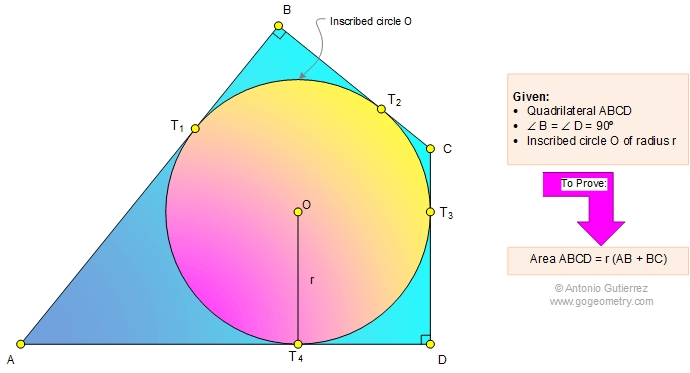# Problem 1097: Quadrilateral, Inscribed Circle, 90 Degree, Angle, Area. Level: High School, SAT Prep, College Geometry

< PREVIOUS PROBLEM  |  NEXT PROBLEM >

 The figure below shows a quadrilateral ABCD and the inscribed circle O (radius r) so that angle B = angle D = 90 degree. Prove that area ABCD = r(AB + BC). Problem submitted by Maurice Ho.Home | Search | Geometry | Problems | All Problems | Open Problems | Visual Index | 1091-1100 | Quadrilaterals | Circle | Tangent Line | Area | Perpendicular lines | Email | Post a comment or solution | By Antonio GutierrezLast updated: Mar 13, 2015